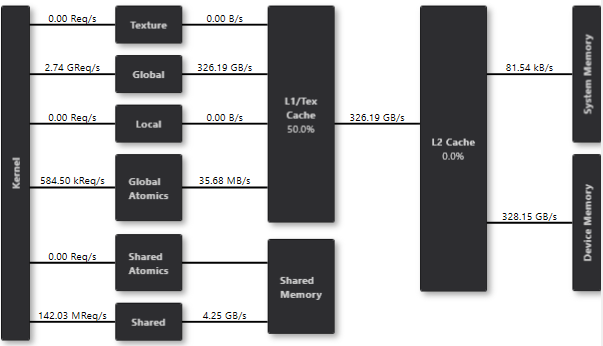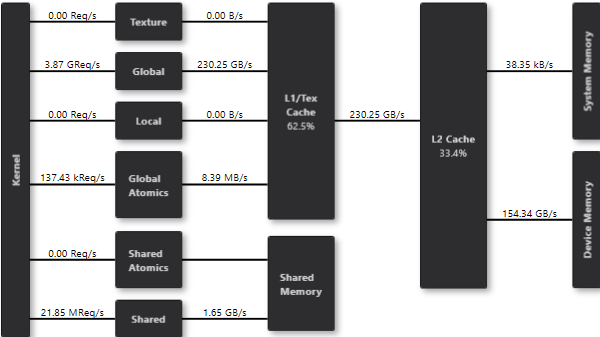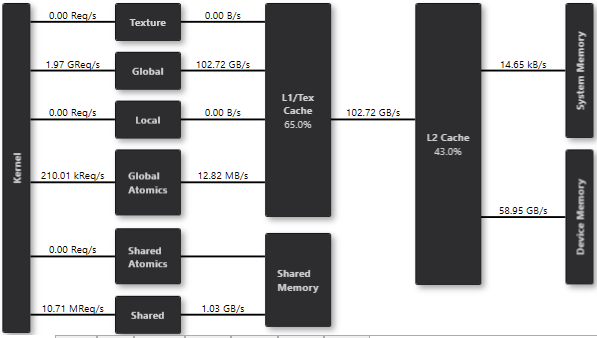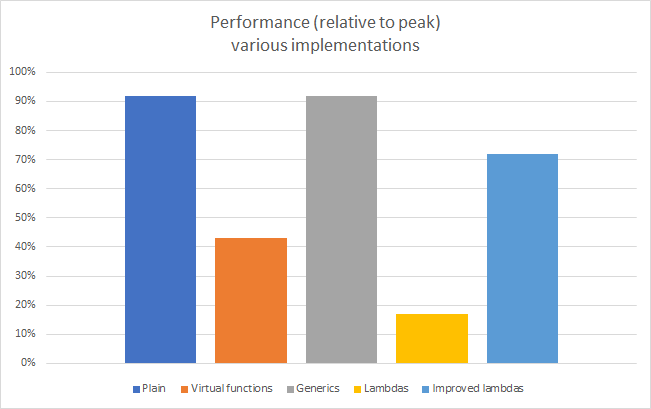### Generics, virtual functions, lambdas, a short guide to performance

Hybridizer supports various “advanced” C# constructs, such as

These features help the user to write expressive and reusable code. But performance wise, they come at a cost. This post will detail these costs, and how to mitigate them.
We performed all performance measurements with a GeForce 1080 Ti, on Windows 10 and using CUDA 10.0 within Visual Studio 2017 community.

## Plain code

``` ```

``` [EntryPoint] public static void ReduceAdd(float N, float[] a, float[] result) { var cache = new SharedMemoryAllocator<float>().allocate(blockDim.x); int tid = threadIdx.x + blockDim.x * blockIdx.x; int cacheIndex = threadIdx.x; float tmp = 0.0F; while (tid < N) { tmp += a[tid]; tid += blockDim.x * gridDim.x; } cache[cacheIndex] = tmp; CUDAIntrinsics.__syncthreads(); int i = blockDim.x / 2; while (i != 0) { if (cacheIndex < i) { cache[cacheIndex] += cache[cacheIndex + i]; } CUDAIntrinsics.__syncthreads(); i >>= 1; } if (cacheIndex == 0) { AtomicExpr.apply(ref result, cache, (x, y) => x + y); } ```

This code can be run using the usual boilerplate:

``` int N = 1024 * 1024 * 32; float[] a = new float[N]; float[] result = new float; var runner = HybRunner.Cuda().Wrap(new PlainReduction()).ReduceAdd(N, a, result); ```

With appropriate blockSize and gridDim, this code delivers 328 GB/s:Bandwidth test (from CUDA samples), shows that peak bandwidth is 355 GB/s on my machine.
That means naïve code reaches 92% of peak.

## Virtual functions

Virtual functions allow user to share piece of code, relying on interfaces, or some inheritance hierarchy instead of concrete implementations. For example, we can write a reduction function working for addition, multiplication, maximum, or user defined code:

``` ```

``` public interface IFunc { [Kernel] float run(float x, float y); } public class AddFunc : IFunc { [Kernel] public float run(float x, float y) { return x + y; } } public class Reduction { [EntryPoint] public static void Run(IFunc func, int N, float[] a, float[] result) { var cache = new SharedMemoryAllocator<float>().allocate(blockDim.x); int tid = threadIdx.x + blockDim.x * blockIdx.x; int cacheIndex = threadIdx.x; float tmp = 0.0F; while (tid < N) { tmp = func.run(tmp, a[tid]); tid += blockDim.x * gridDim.x; } // ... } ```

As detailed in documentation, this code generates dispatching functions.
That means that for every call to ‘func.run’, we look at ‘func’ type id to get the right implementation and then run it. This lookup is cached in the L1/Tex cache of the GPU, but still generates memory dependency, and therefore impacts performance.

This version yields at best 154GB/s, a disappointing 43% of peak bandwidth:It’s really important to note that a virtual function table cannot be inlined. Indeed, compiler don’t know the typeid before the code is actually running.

## Generics

Performance can be restored using generics. Indeed, Hybridizer maps them to C++ templates. C++ templates are resolved at compile time. Therefore, we need to tell Hybridizer which actual types will be used at runtime. That can be done through attributes:

``` ```

``` [HybridTemplateConcept] public interface IFunc { [Kernel] float run(float x, float y); } public struct AddFunc : IFunc { [Kernel] public float run(float x, float y) { return x + y; } } [HybridRegisterTemplate(Specialize = typeof(Reduction<AddFunc>))] public class Reduction<T> where T: struct, IFunc { [Kernel] T reductor { get; set; } public Reduction() { reductor = default(T); } [Kernel] public void Run(int N, float[] a, float[] result) { var cache = new SharedMemoryAllocator<float>().allocate(blockDim.x); int tid = threadIdx.x + blockDim.x * blockIdx.x; int cacheIndex = threadIdx.x; float tmp = 0.0F; while (tid < N) { tmp = reductor.run(tmp, a[tid]); tid += blockDim.x * gridDim.x; } // ... } ```

Hybridizer doesn’t support generic entrypoints. That’s because all generated code must be properly specialized in order to get marshalled at runtime. We therefore need to wrap actual code in a specialized entrypoint:

``` ```

``` public class EntryPoints { [EntryPoint] public static void ReduceAdd(Reduction<AddFunc> reductor, int N, float[] a, float[] result) { reductor.Run(N, a, result); } } ```

This code is a bit heavier than the “interfaces” version. However, it delivers the same performance as the “plain” version. Here is a profiling with various block/thread sizes for the three versions :## Delegates and Lambdas

Another way to get expressive and reusable code in C# are Delegates or, since C# 2.0, lambdas. As discussed in documentation, delegates can be provided at runtime. This breaks any possibility of inlining, and has extreme cost in performance.

``` ```

``` public class Reduction { Func<float, float, float> localReductor; public Reduction(Func<float, float, float> func) { localReductor = func; } [Kernel] public void Reduce(int N, float[] a, float[] result) { var cache = new SharedMemoryAllocator<float>().allocate(blockDim.x); int tid = threadIdx.x + blockDim.x * blockIdx.x; int cacheIndex = threadIdx.x; float tmp = 0.0F; while (tid < N) { tmp = localReductor(tmp, a[tid]); tid += blockDim.x * gridDim.x; } // ... } } ```

This code delivers 59GB/s, which is only 17% of peak:As such, runtime lambda are quite a trap!

Fortunately, there is a way to get some performance back. If you save the localReductor in a temporary variable (for each thread), it will be stored in registers. And then nvcc is able to do some magic.

``` ```

``` public class Reduction { Func<float, float, float> localReductor; public Reduction(Func<float, float, float> func) { localReductor = func; } [Kernel] public void Reduce(int N, float[] a, float[] result) { Func<float, float, float> f = localReductor; // !!! var cache = new SharedMemoryAllocator<float>().allocate(blockDim.x); int tid = threadIdx.x + blockDim.x * blockIdx.x; int cacheIndex = threadIdx.x; float tmp = 0.0F; while (tid < N) { tmp = f(tmp, a[tid]); tid += blockDim.x * gridDim.x; } // ... } } ```

and the achieved bandwidth is now back at high levels, at 255GB/s, 72% of peak.

## Summary

There are various ways to write expressive and reusable code. However, when it comes to the GPU, they can have very high to very little (if any) impact on performances.